# A Starbase Guide

## Fuel Time - YOLOL Script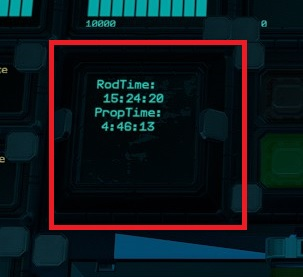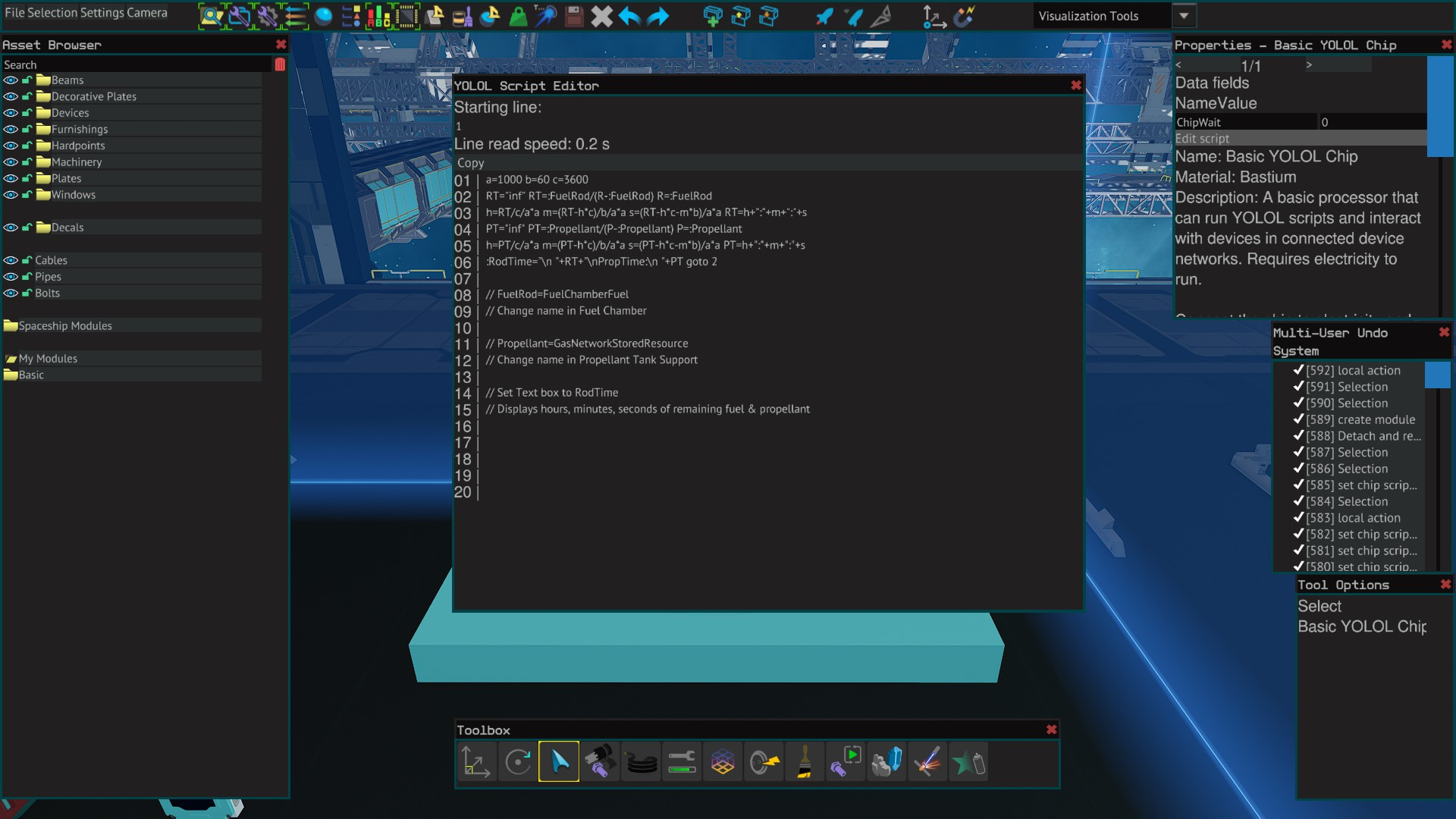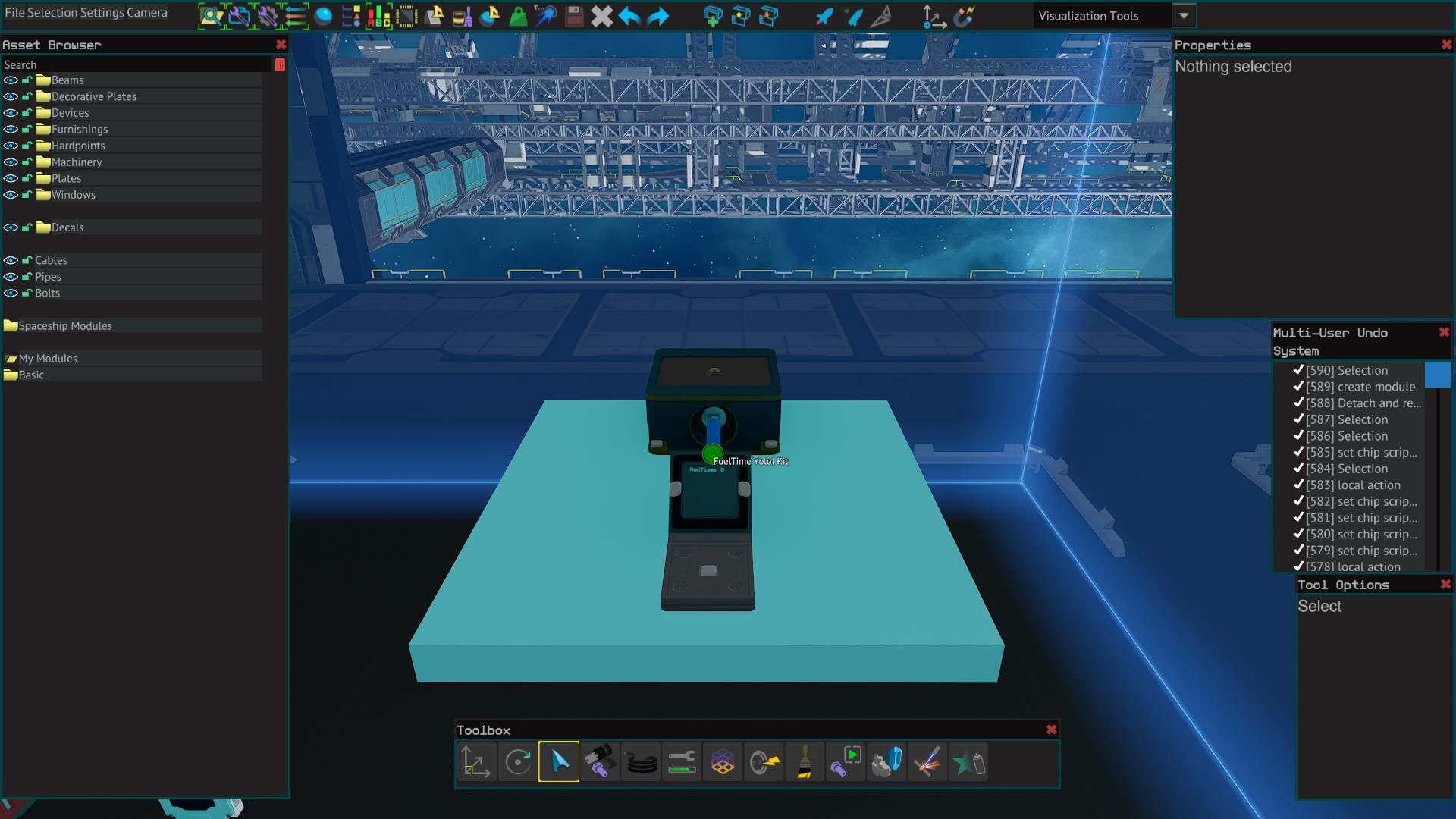Displays the remaining time in hours, minutes and seconds of both your fuel rods and your propellant on a 24x24 text display.

Requires:

1x Basic YOLOL Chip

1x YOLOL Chip Socket or 1x YOLOL Rack Chip Slot

1x Text Panel 24x24cm

YOLOL Code:

YOLOL Code - copy & paste version:

a=1000 b=60 c=3600 RT="inf" RT=:FuelRod/(R-:FuelRod) R=:FuelRod h=RT/c/a*a m=(RT-h*c)/b/a*a s=(RT-h*c-m*b)/a*a RT=h+":"+m+":"+s PT="inf" PT=:Propellant/(P-:Propellant) P=:Propellant h=PT/c/a*a m=(PT-h*c)/b/a*a s=(PT-h*c-m*b)/a*a PT=h+":"+m+":"+s :RodTime="\n "+RT+"\nPropTime:\n "+PT goto 2 // FuelRod=FuelChamberFuel // Change name in Fuel Chamber // Propellant=GasNetworkStoredResource // Change name in Propellant Tank Support // Set Text box to RodTime // Displays hours, minutes, seconds of remaining fuel & propellant (ctrl+c=copy, ctrl+v=paste)

Installation

Copy and paste the yolol code to the basic chip 1 line at a time.

Mount chip in a powered chip socket or in a spare rack slot.

Rename FuelRod in the script to whatever your fuelchamberfuel is named.

Alternatively change the FuelChamberFuel of the fuel chamber to FuelRod.

Rename Propellant in the script to whatever your GasNetworkStoredResource is named.

Alternatively change the GasNetworkStoredResource of the Propellant Tank Support to Propellant.

Place the 24x24 text panel and change PanelValue to RodTime.

FuelTime Yolol Kit

## Dual Laser Pulse - YOLOL Script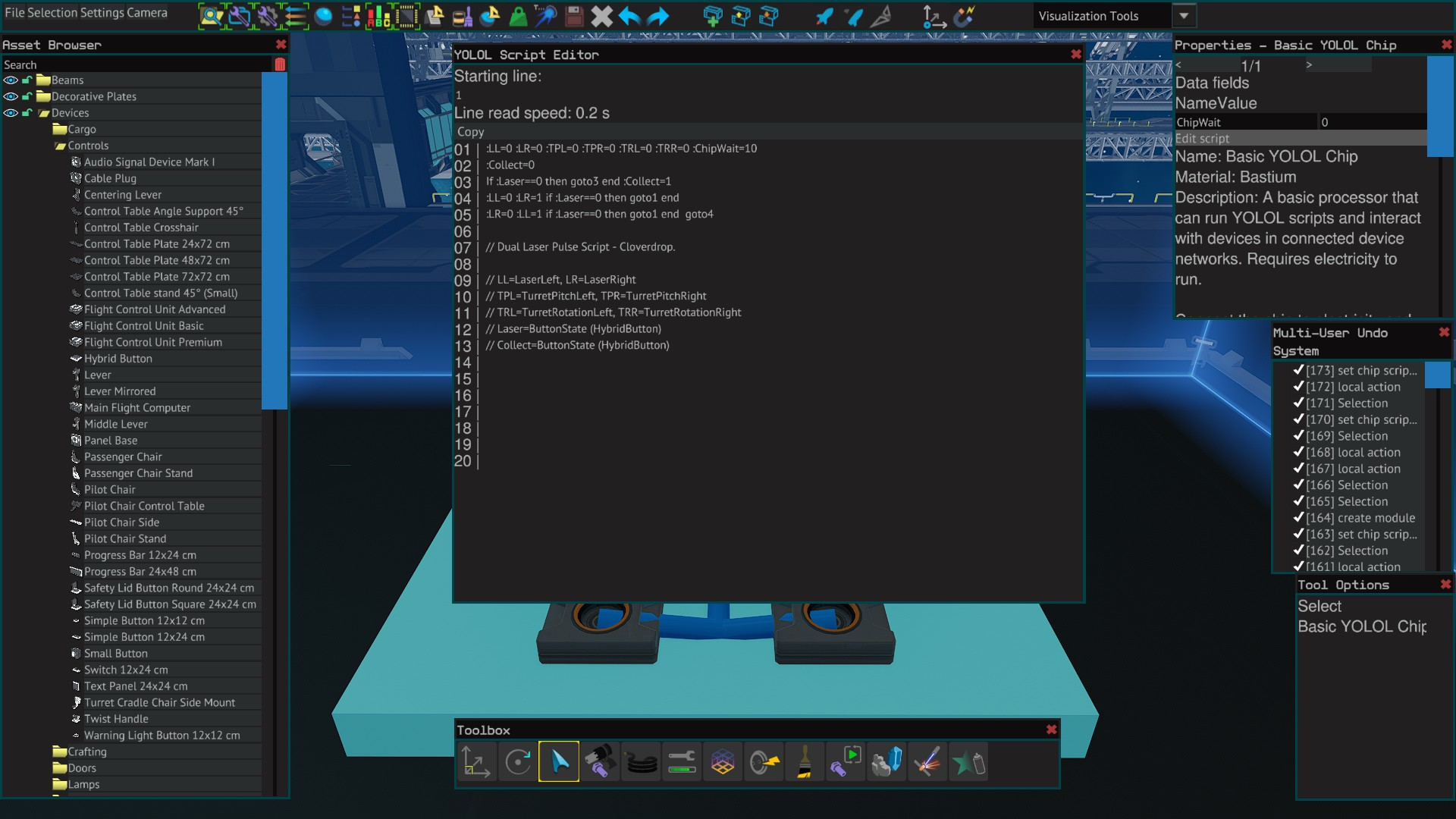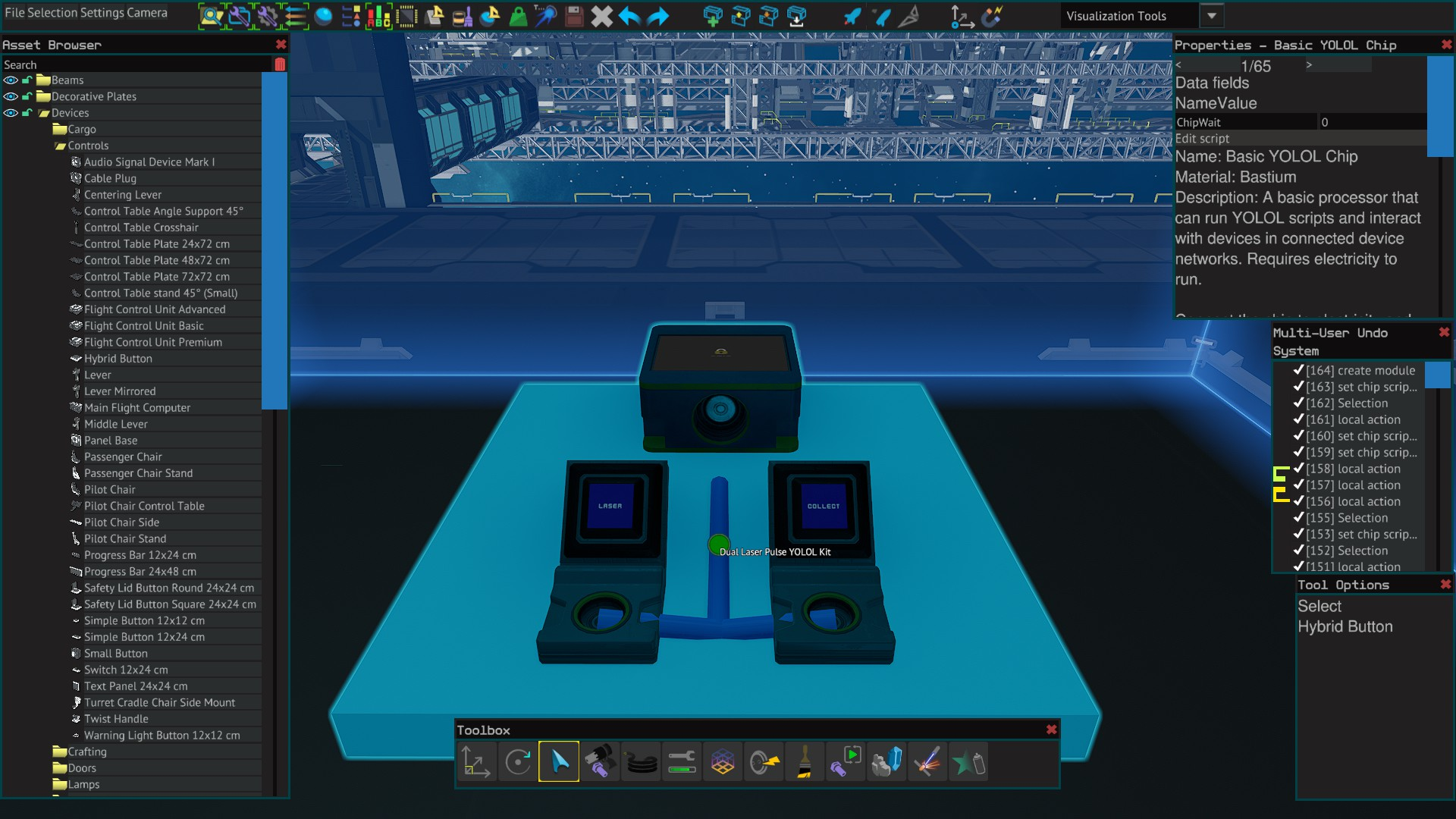A pulse script for dual mining lasers.

Requires:

1x Basic YOLOL Chip

1x YOLOL Chip Socket or 1x YOLOL Rack Chip Slot

2x Hybrid Button

YOLOL Code:

Dual Pulse Basic YOLOL Code - copy & paste version:

:LL=0 :LR=0 :TRL=0 :TPR=0 :TRL=0 :TRR=0 :ChipWait=10 :Collect=0 If :Laser==0 then goto3 end :collect=1 :LL=0 :LR=1 if :Laser==0 then goto1 end :LL=1 :LR=0 if :Laser==0 then goto1 end goto 4 // Dual Laser Pulse Script - Cloverdrop. // LL=LaserLeft, LR=LaserRight // TPL=TurretPitchLeft, TPR=TurretPitchRight // TRL=TurretRotationLeft, TRR=TurretRotationRight // Laser=ButtonState (HybridButton) // Collect=ButtonState (HybridButton) (ctrl+c=copy, ctrl+v=paste)

Installation

Copy and paste the yolol code to the basic chip 1 line at a time.

Mount chip in a powered chip socket or in a spare rack slot.

Rename LL, LR, TPL, TPR, TRL, TRR in the script to whatever your lasers, TurretPitch and TurretRotation fields are named as.

Alternatively change the field names of the lasers, pitch and rotation to match those in the script.

Place 2 hybrid buttons and rename the ButtonState fields to Laser, Collect

Change ChipWait value to auto shut off the collector after desired amount of seconds. (1=0.2 seconds, 5=1 second, 10=2, etc.)

Dual Laser Pulse Yolol Kit

## Dual Sweep & Pulse - YOLOL Script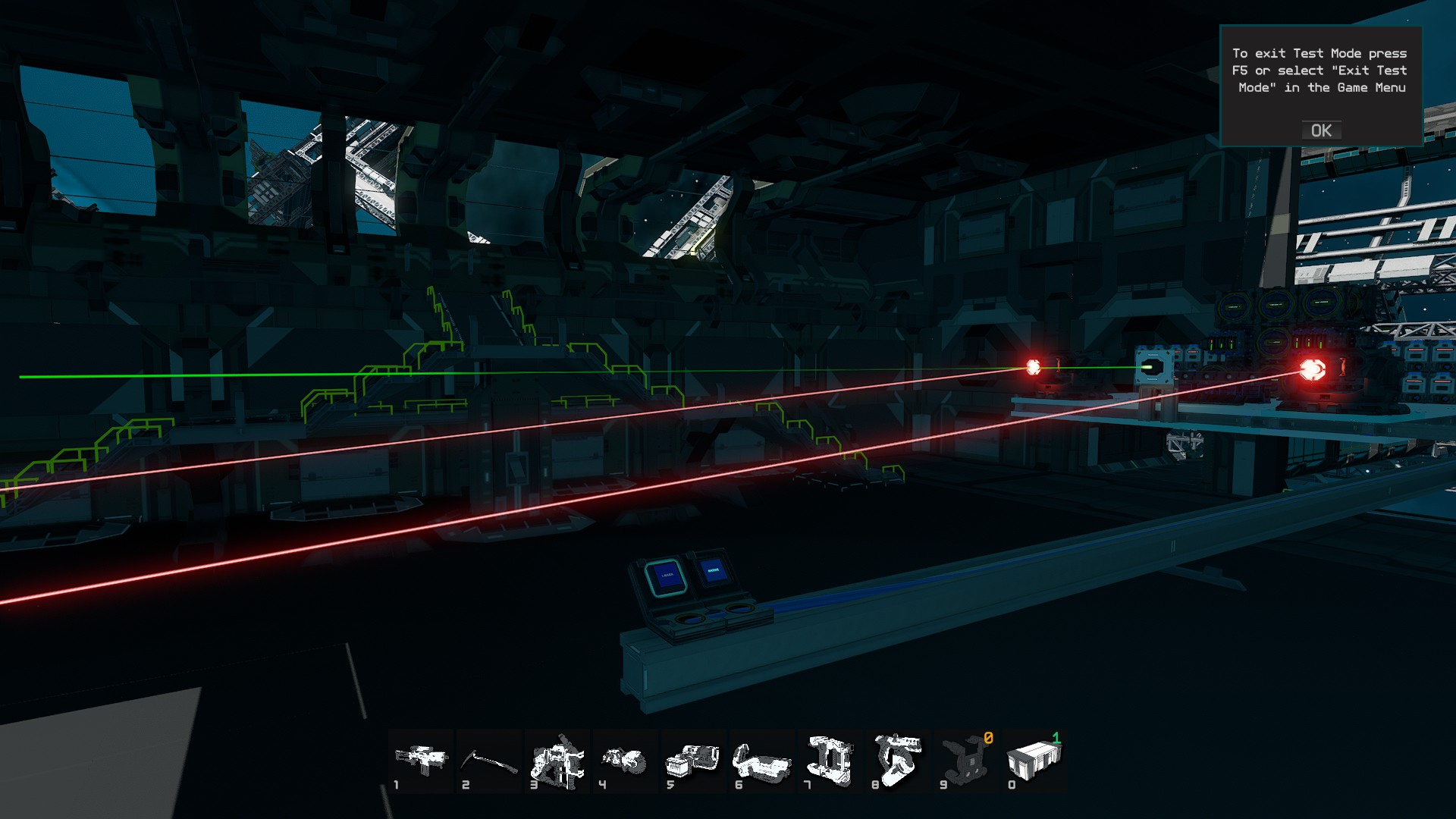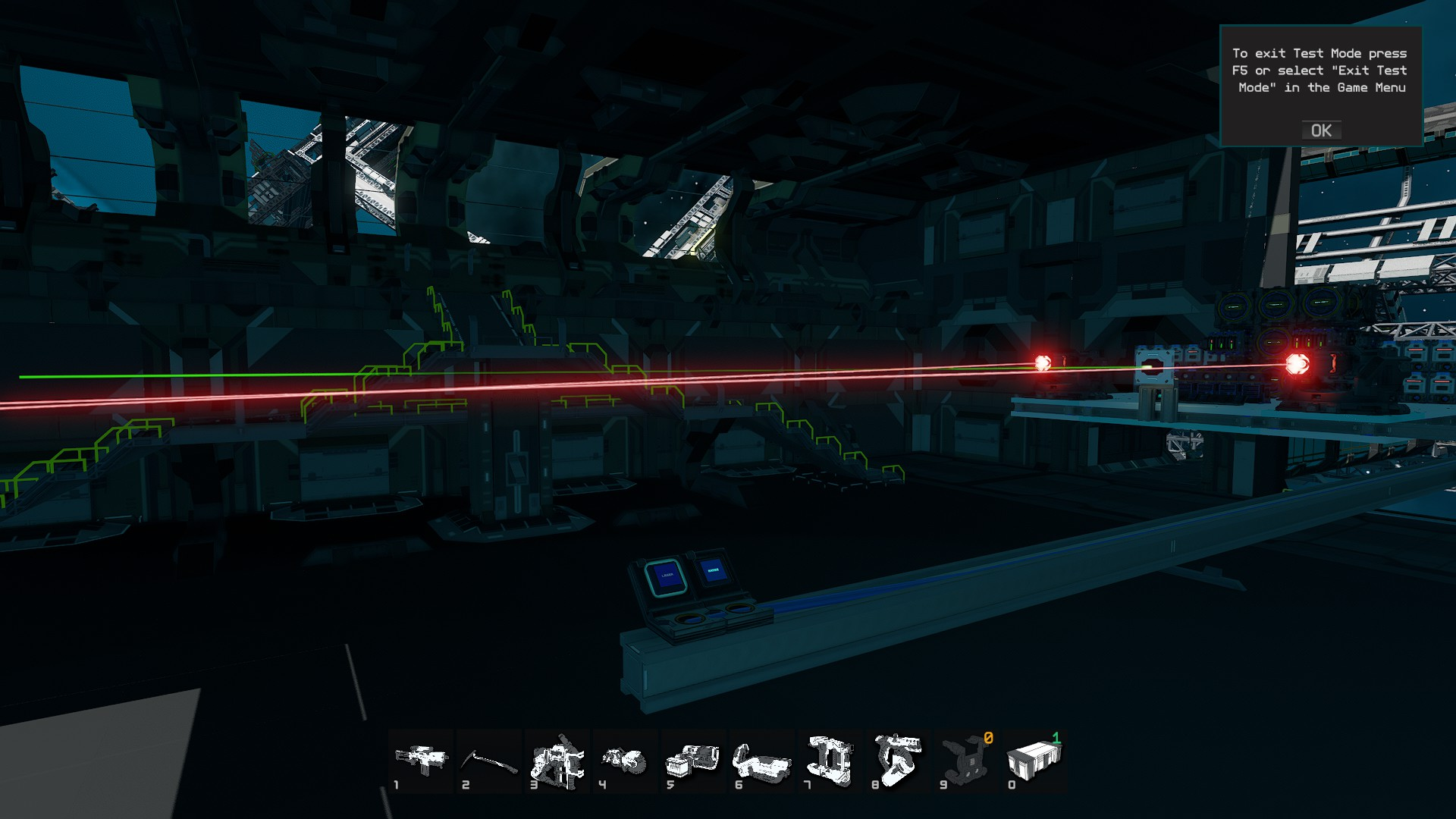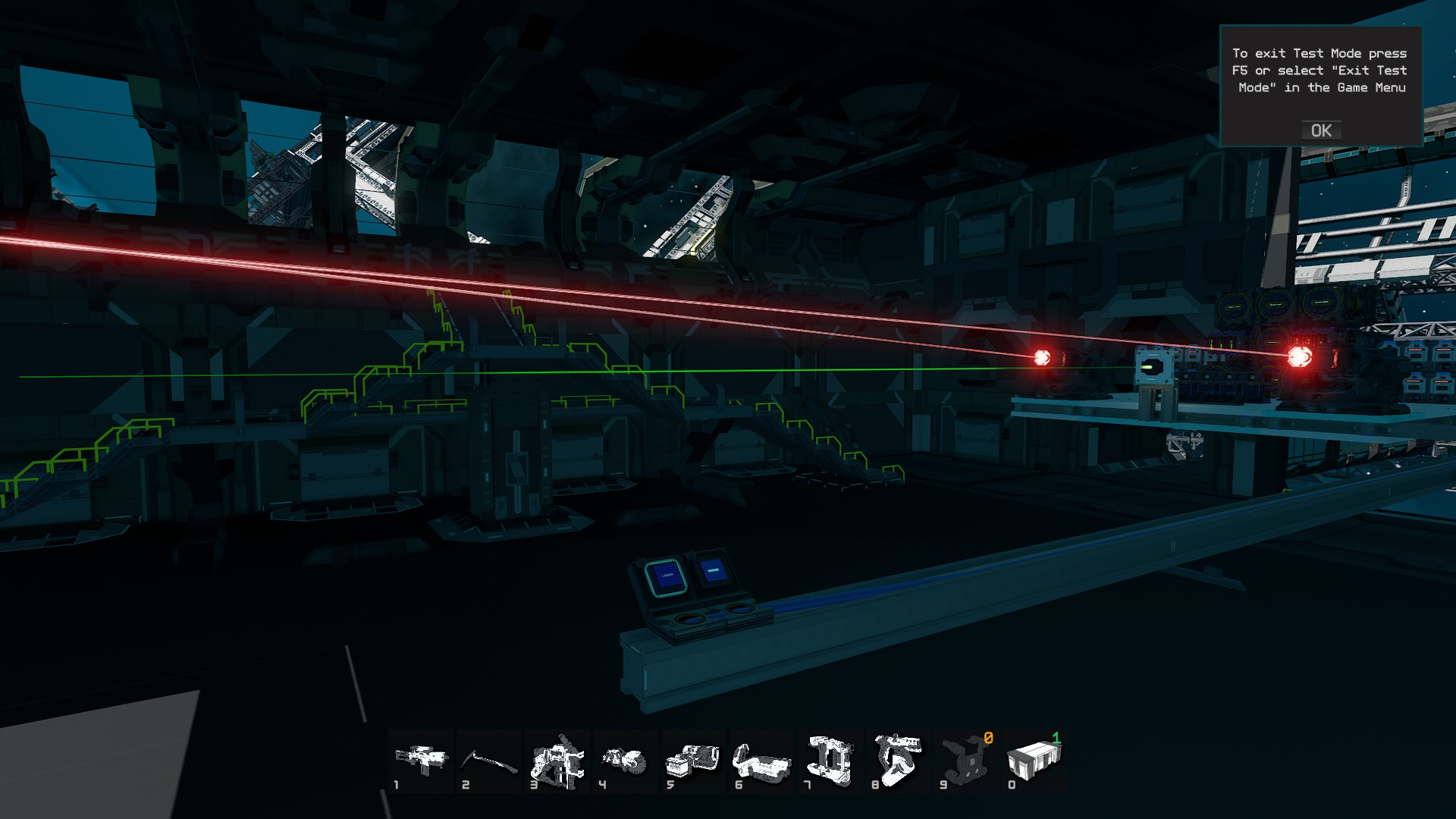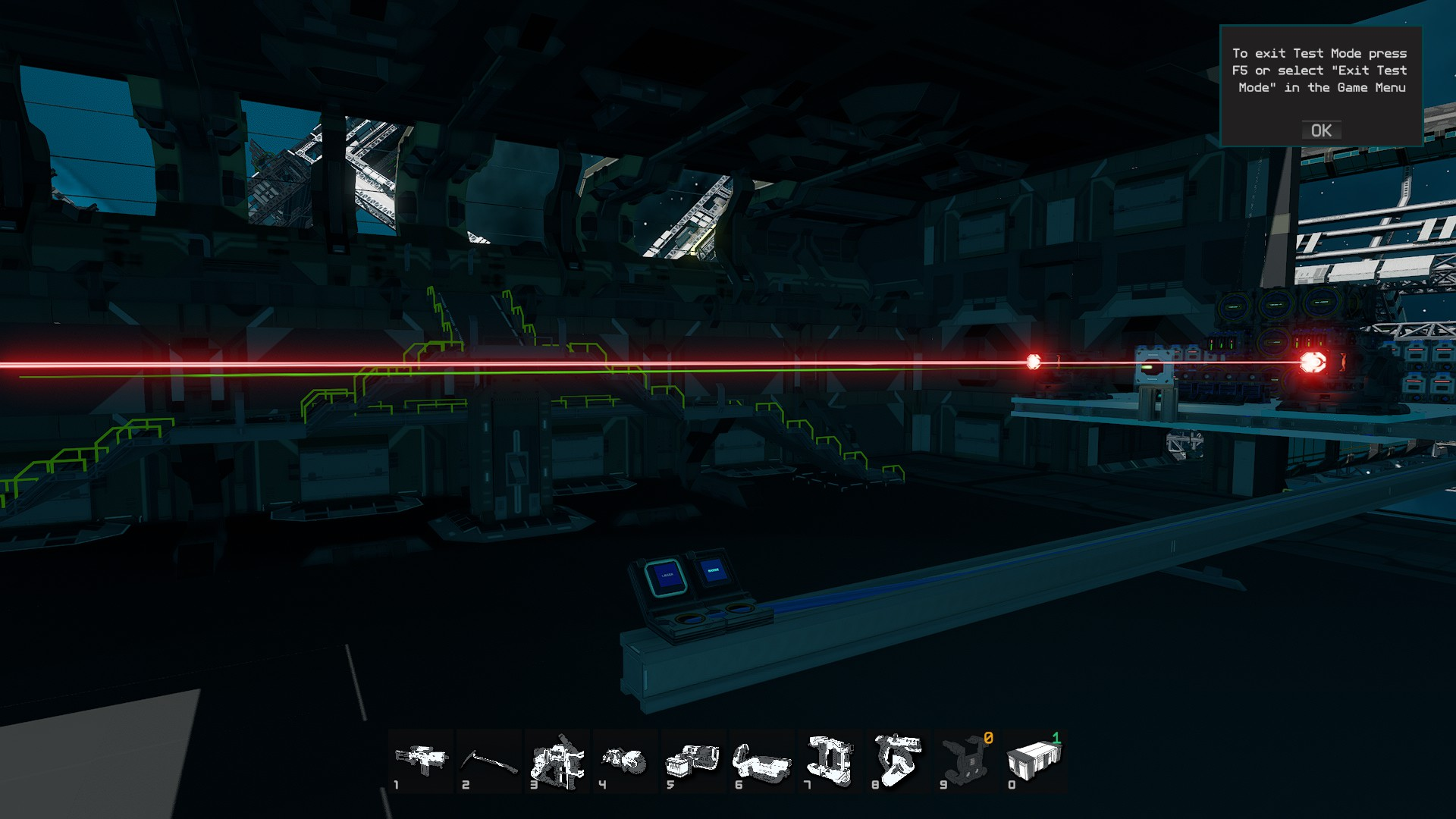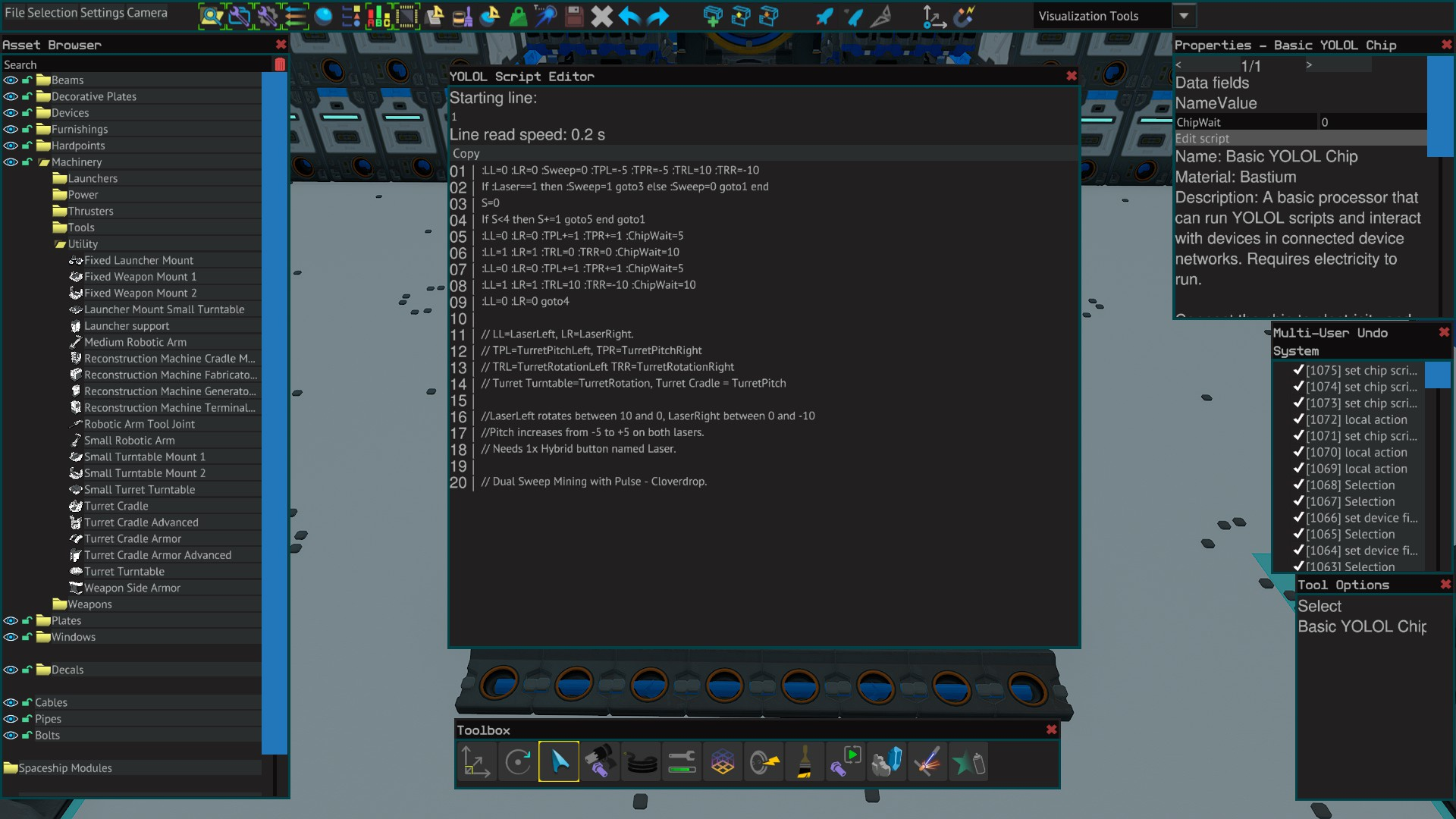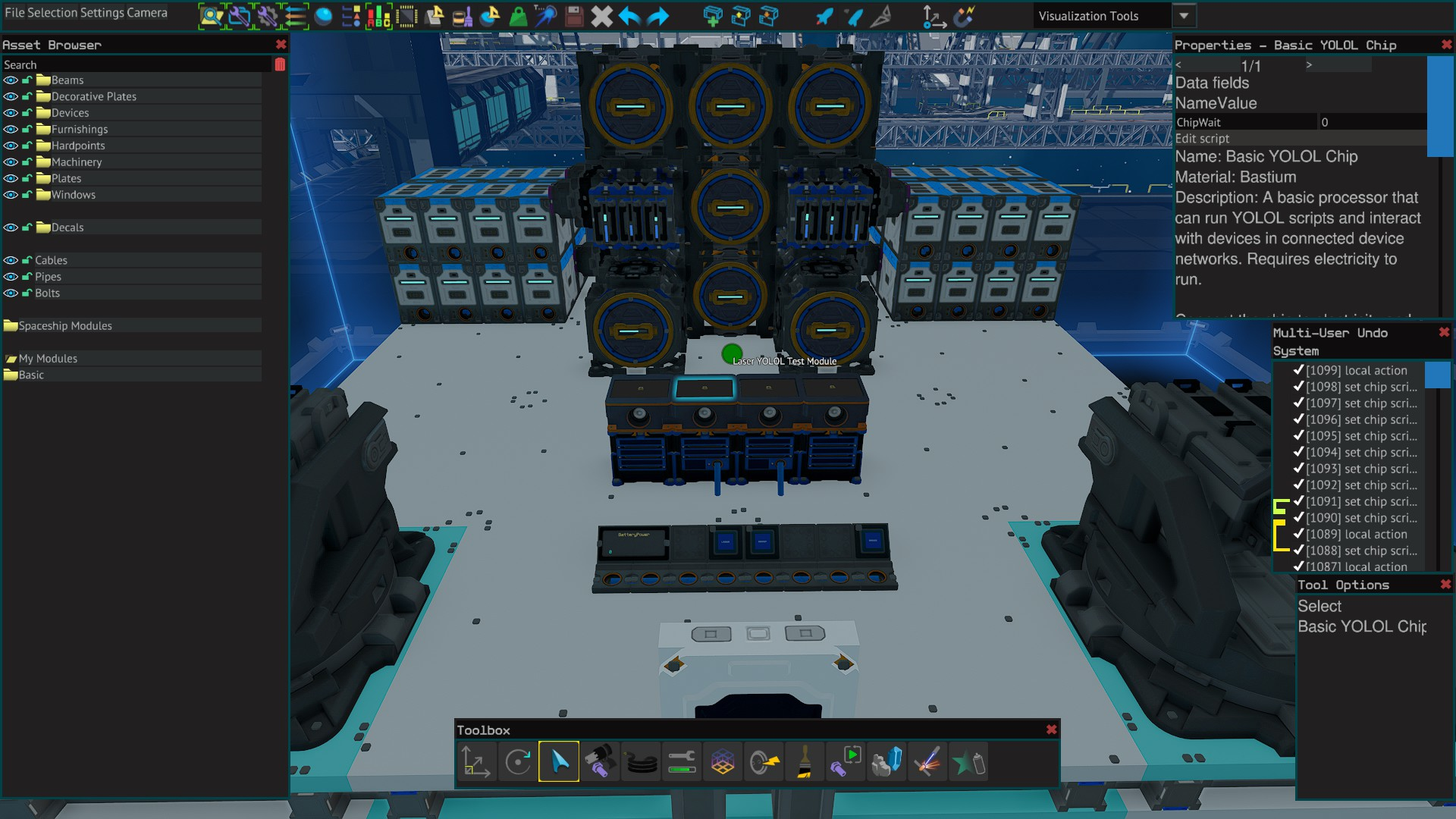A setup for 2x mining laser to sweep through rotation and pitch a number of times while pulsing on and off when not sweeping across the asteroid.

Requires:

1x Basic YOLOL Chip

1x YOLOL Chip Socket or 1x YOLOL Rack Chip Slot

1x Hybrid ButtonYOLOL Code:

Basic YOLOL Code - copy & paste version:

:LL=0 :LR=0 :Sweep=0 :TPL=-5 :TPR=-5 :TRL=10 :TRR=-10 If :Laser==1 then :Sweep=1 goto5 else :Sweep=0 goto1 end S=0 If S<4 then S+=1 goto5 end goto1 :LL=0 :LR=0 :TPL+=1 :TPR+=1 :ChipWait=5 :LL=1 :LR=1 :TRL=0 :TRR=0 :ChipWait=10 :LL=0 :LR=0 :TPL+=1 :TPR+=1 :ChipWait=5 :LL=1 :LR=1 :TRL=10 :TRR=-10 :ChipWait=10 :LL=0 :LR=0 goto4 // LL=LaserLeft, LR=LaserRight. // TPL=TurretPitchLeft, TPR=TurretPitchRight // TRL=TurretRotationLeft TRR=TurretRotationRight // Turret Turntable=TurretRotation, Turret Cradle = TurretPitch // LaserLeft rotates between 10 and 0, LaserRight between 0 and -10 // Pitch increases from -5 to +5 on both lasers. // Needs 1x Hybrid button named Laser. // Dual Sweep Mining with Pulse - Cloverdrop. (ctrl+c=copy, ctrl+v=paste)

Installation

Copy and paste the yolol code to the basic chip 1 line at a time.

Mount chip in a powered chip socket or in a spare rack slot.

Rename LL, LR, TPL, TPR, TRL, TRR in the script to whatever your lasers, TurretPitch and TurretRotation fields are named as.

Alternatively change the field names of the lasers, pitch and rotation to match those in the script.

Place a hybrid button and rename the ButtonState fields to Laser.

Optional to place a second hybrid button with ButtonState field named Sweep.

Change TurretPitch/Rotation script values to suit your laser positions

ChipWait values = 1=0.2 seconds, 5=1 second, 10=2, etc.

Laser Yolol Test Module

## Ore Scanner - YOLOL Script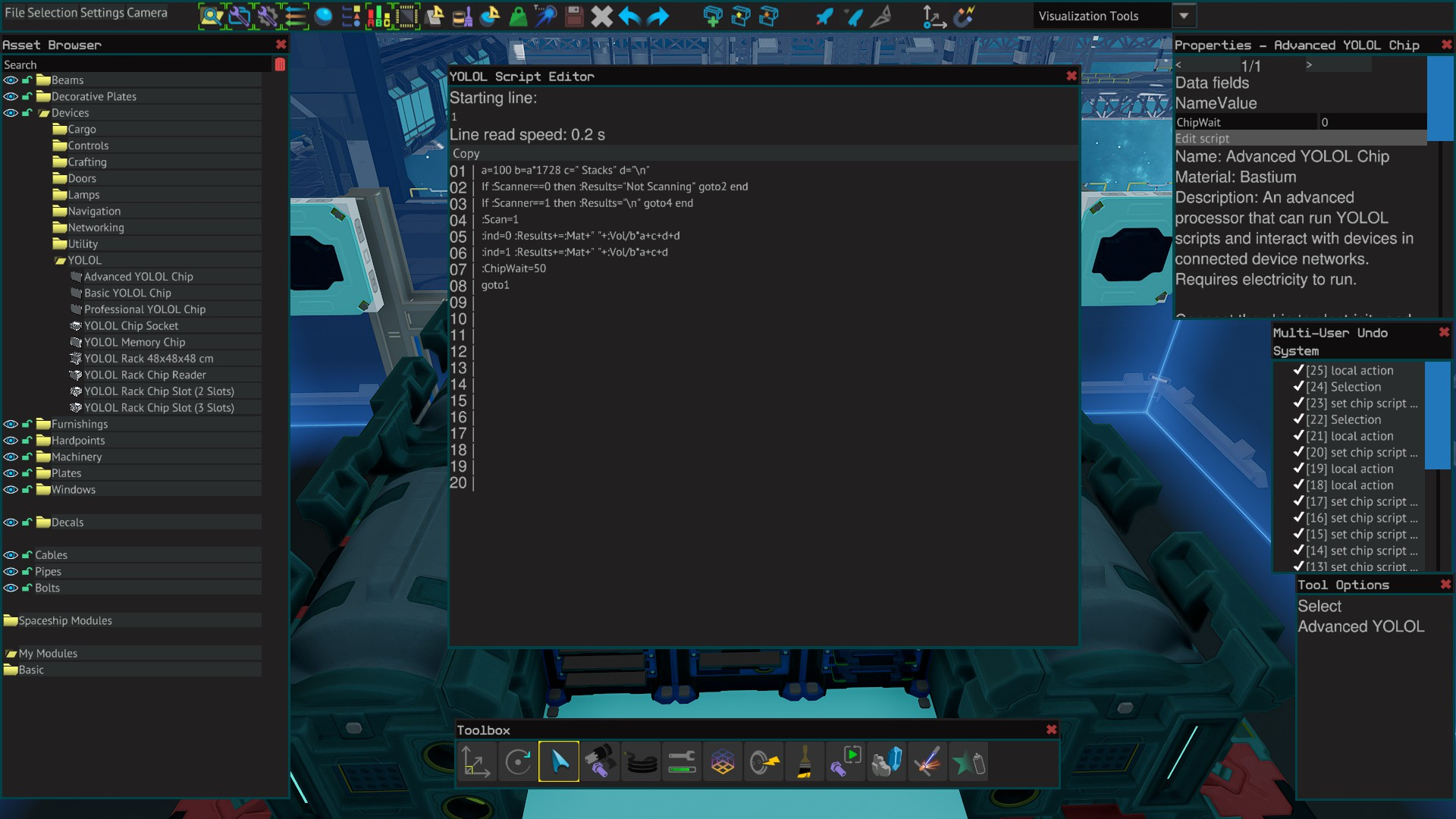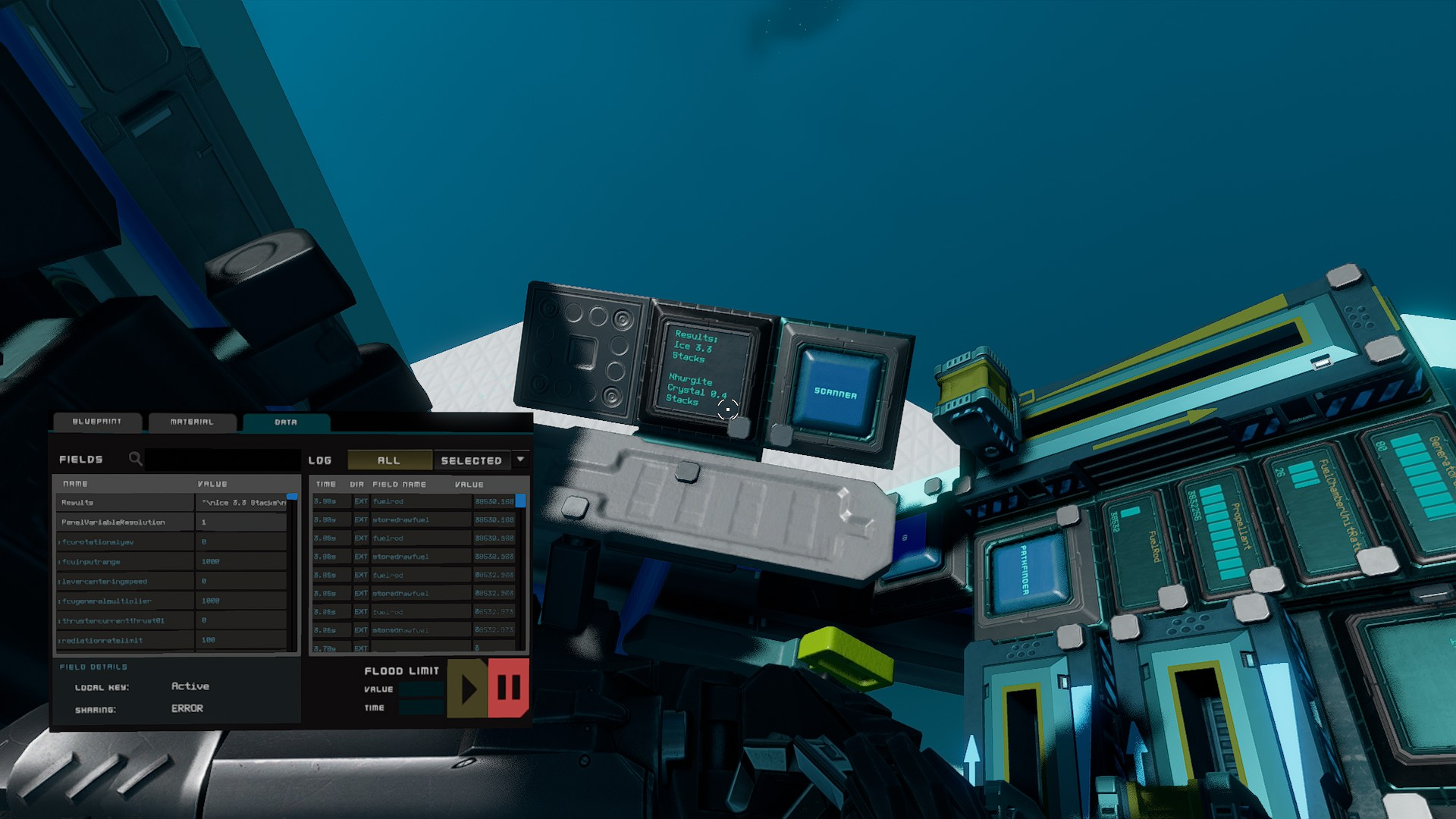The material scanner casts a ray 100 meters long.

If an asteroid is detected within this range, it will update a text panel with the ore type and the amount in stacks.

Requires:

1x YOLOL Chip Socket or 1x YOLOL Rack Chip Slot

1x Text Panel 24x24cm

1x Hybrid Button

YOLOL Code:

Advanced YOLOL Code - copy & paste version:

a=100 b=a*1728 c=" Stacks" d="\n" If :Scanner==0 then :Results="Not Scanning" goto2 end If :Scanner==1 then :Results="\n" goto4 end :Scan=1 :ind=0 :Results+=:Mat+" "+:Vol/b*a+c+d+d :ind=1 :Results+=:Mat+" "+:Vol/b*a+c+d :ChipWait=50 goto1 (ctrl+c=copy, ctrl+v=paste)

Installation

Copy and paste the yolol code to the advanced chip 1 line at a time.

Mount chip in a powered chip socket or in a spare rack slot.

Rename the Material Point Scanner fields as follows ScannerON=Scanner, Index=Ind, Material=Mat, Volume=Vol.

Place the 24x24 text panel and change PanelValue to Results.

Place the Hybrid Button and change ButtonState field to Scanner.

Yolol Kit

## ISAN Waypoints - YOLOL Script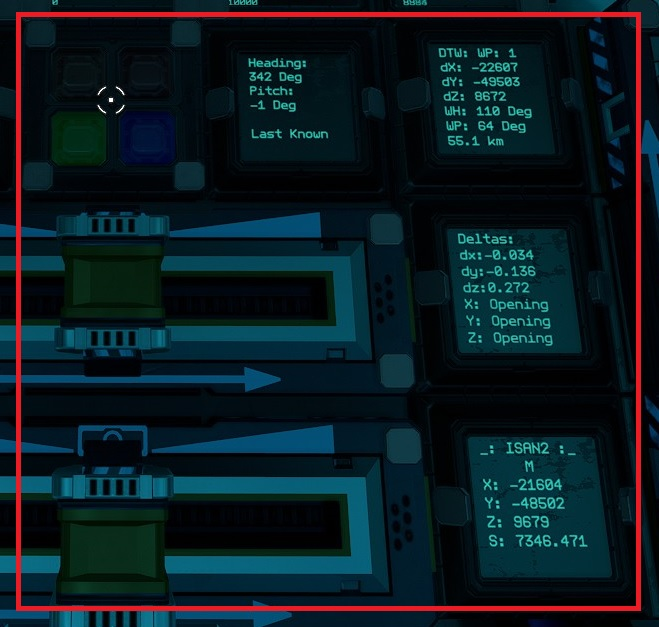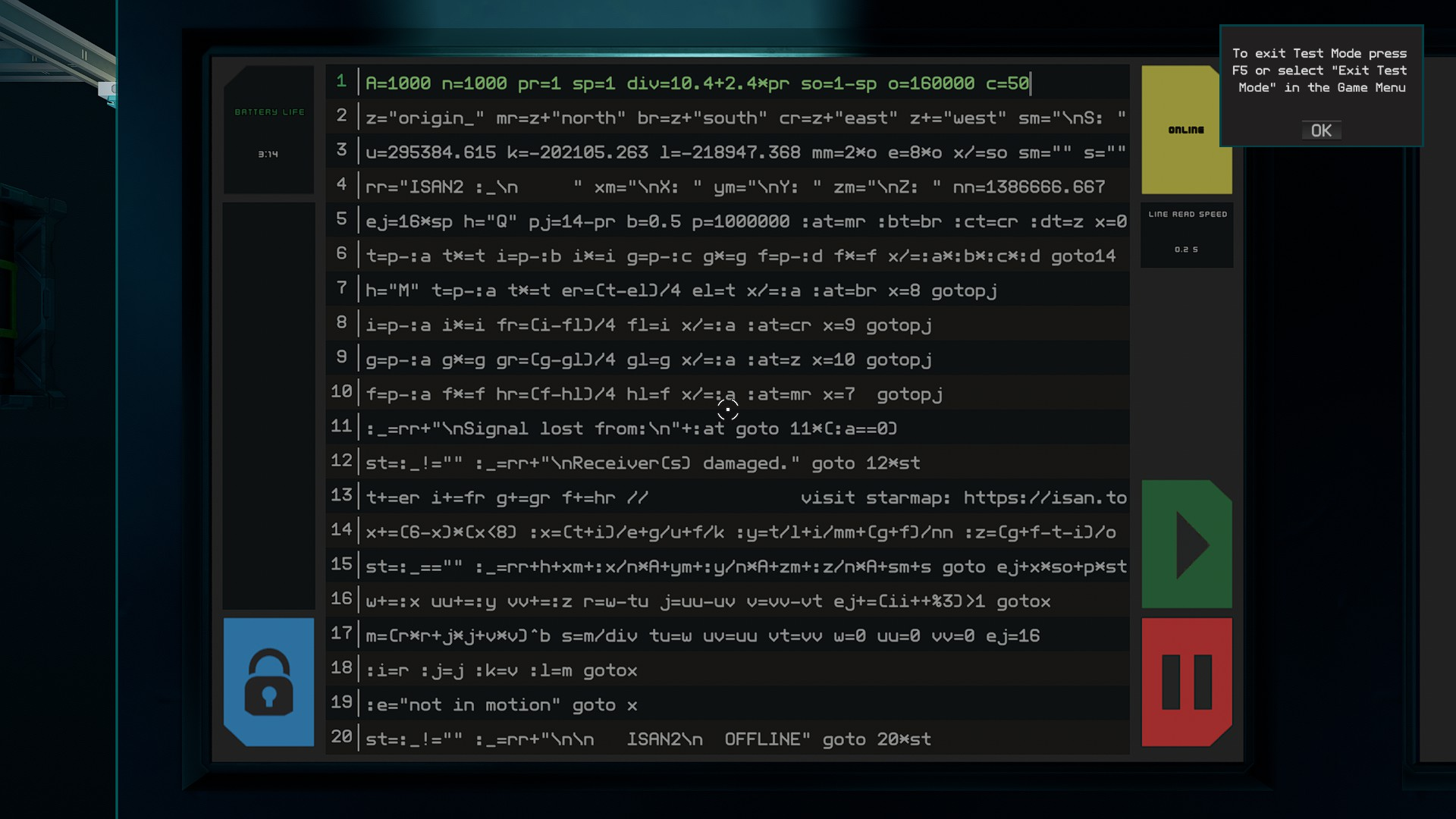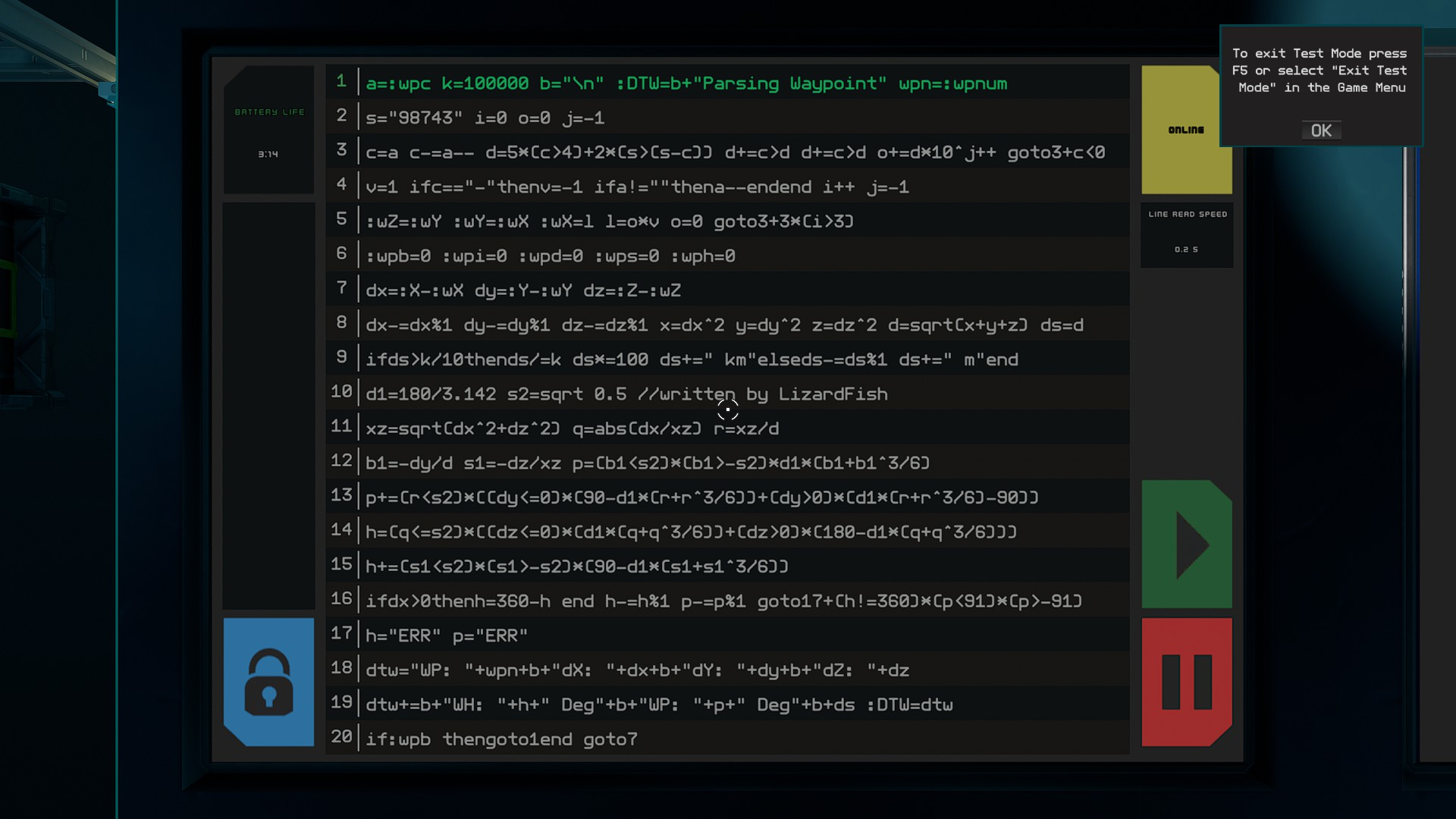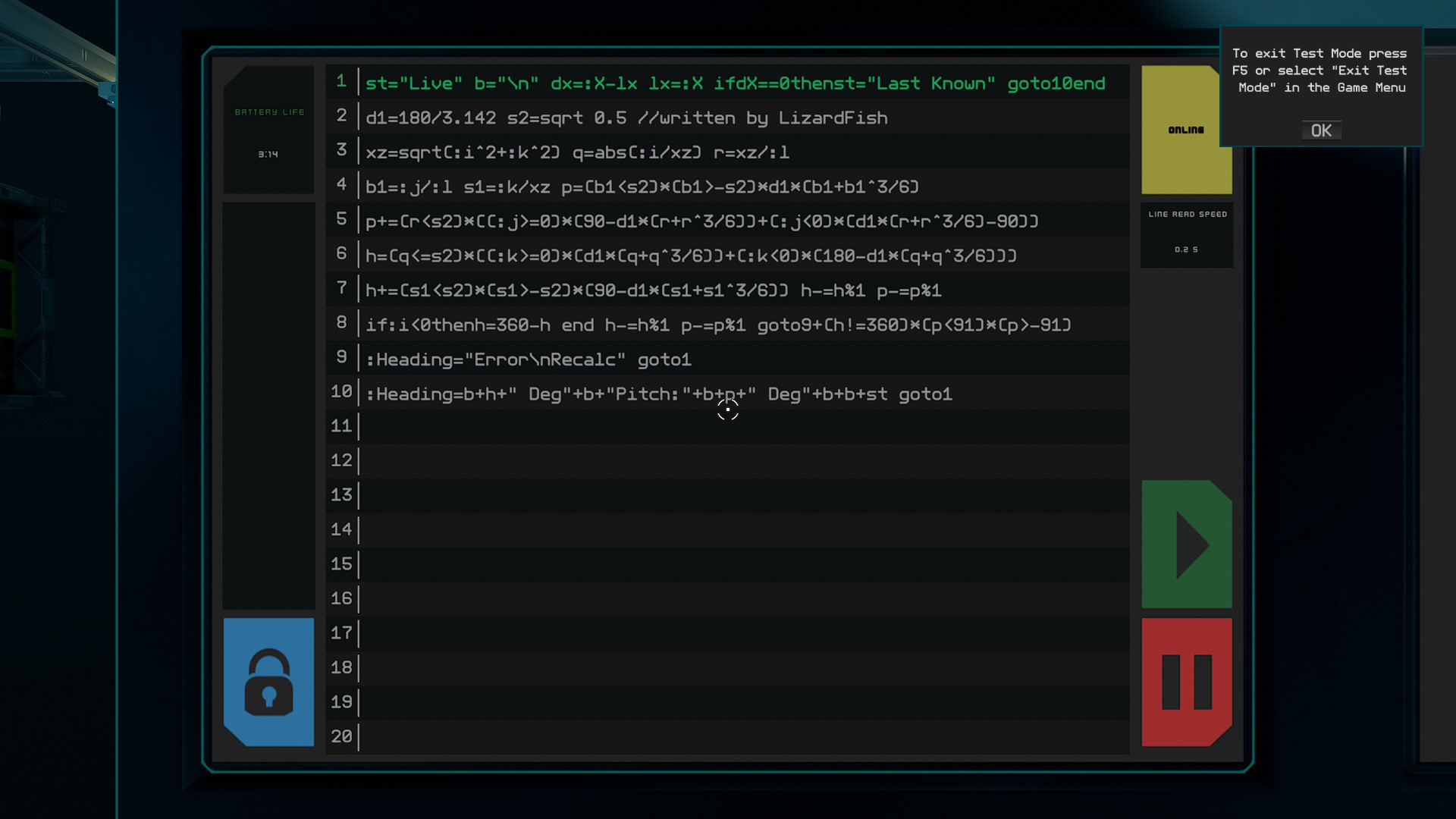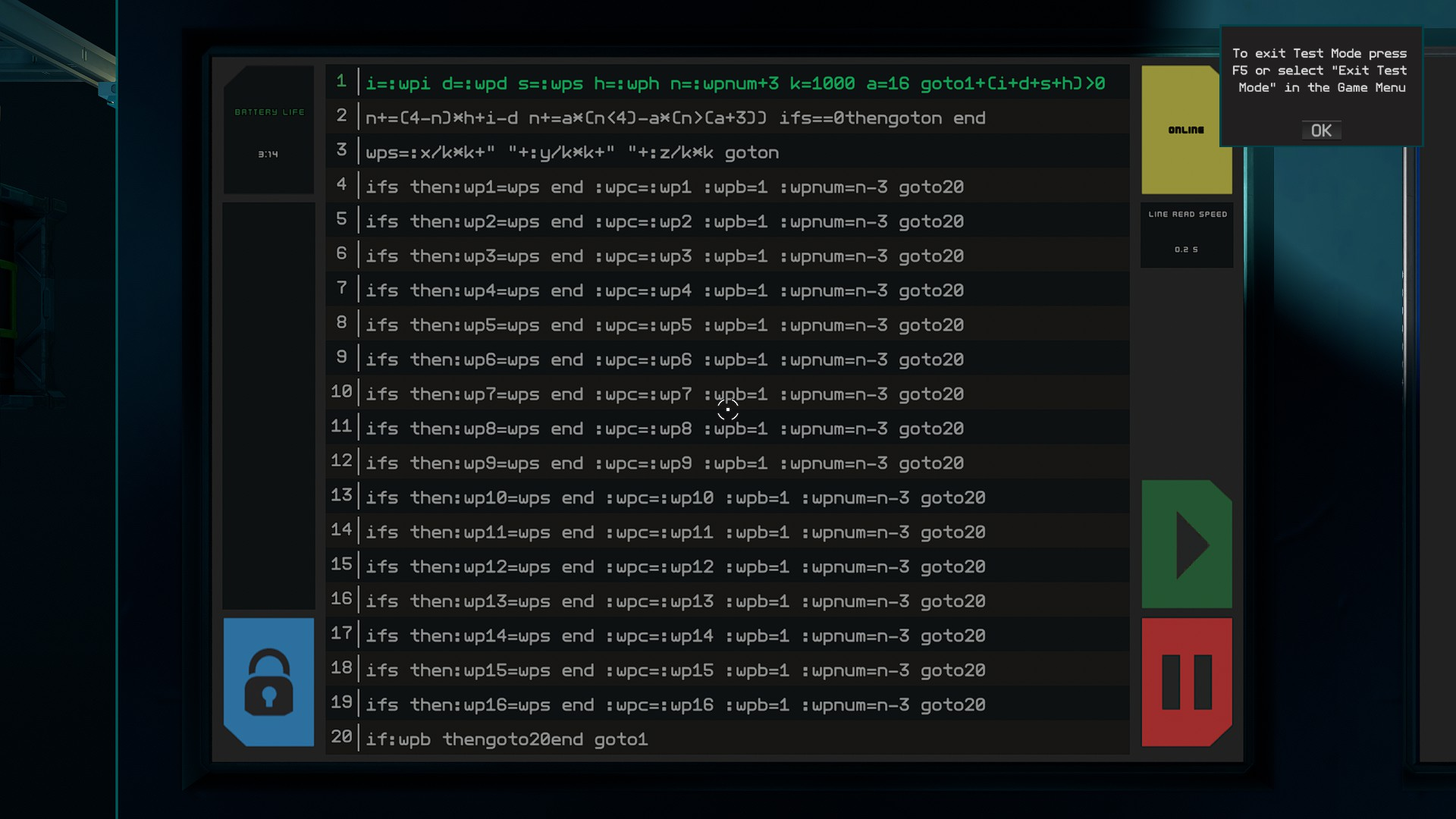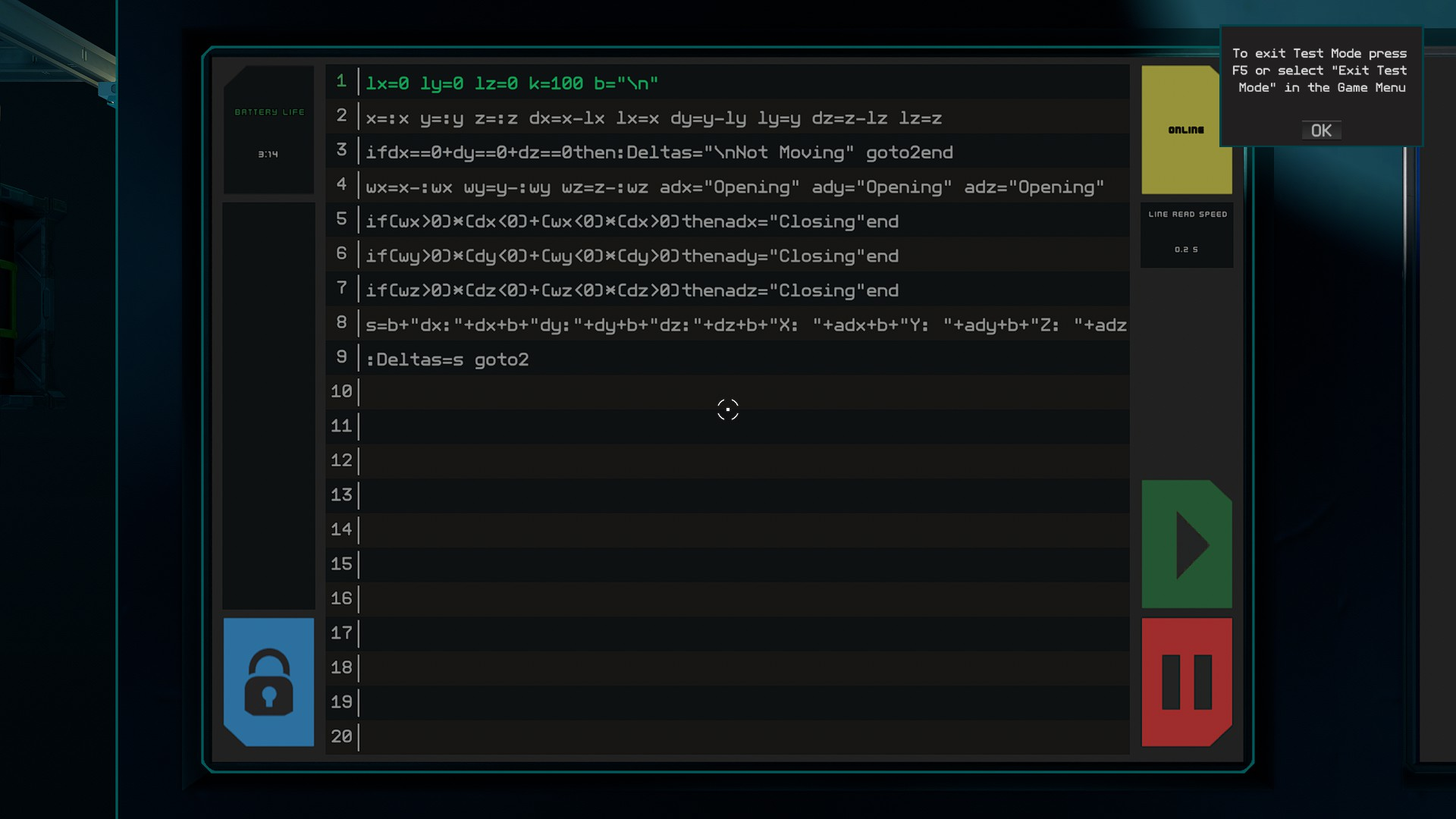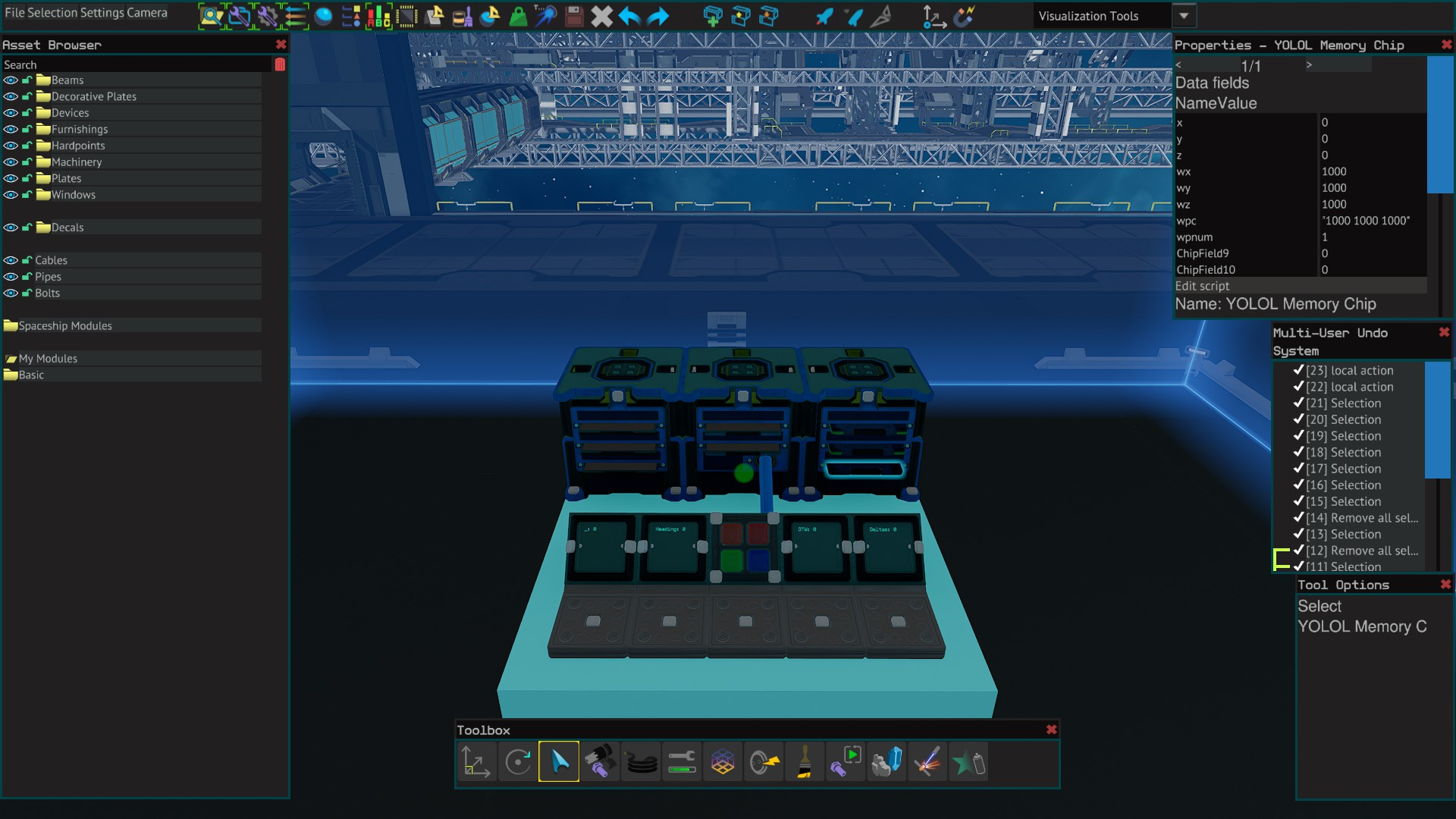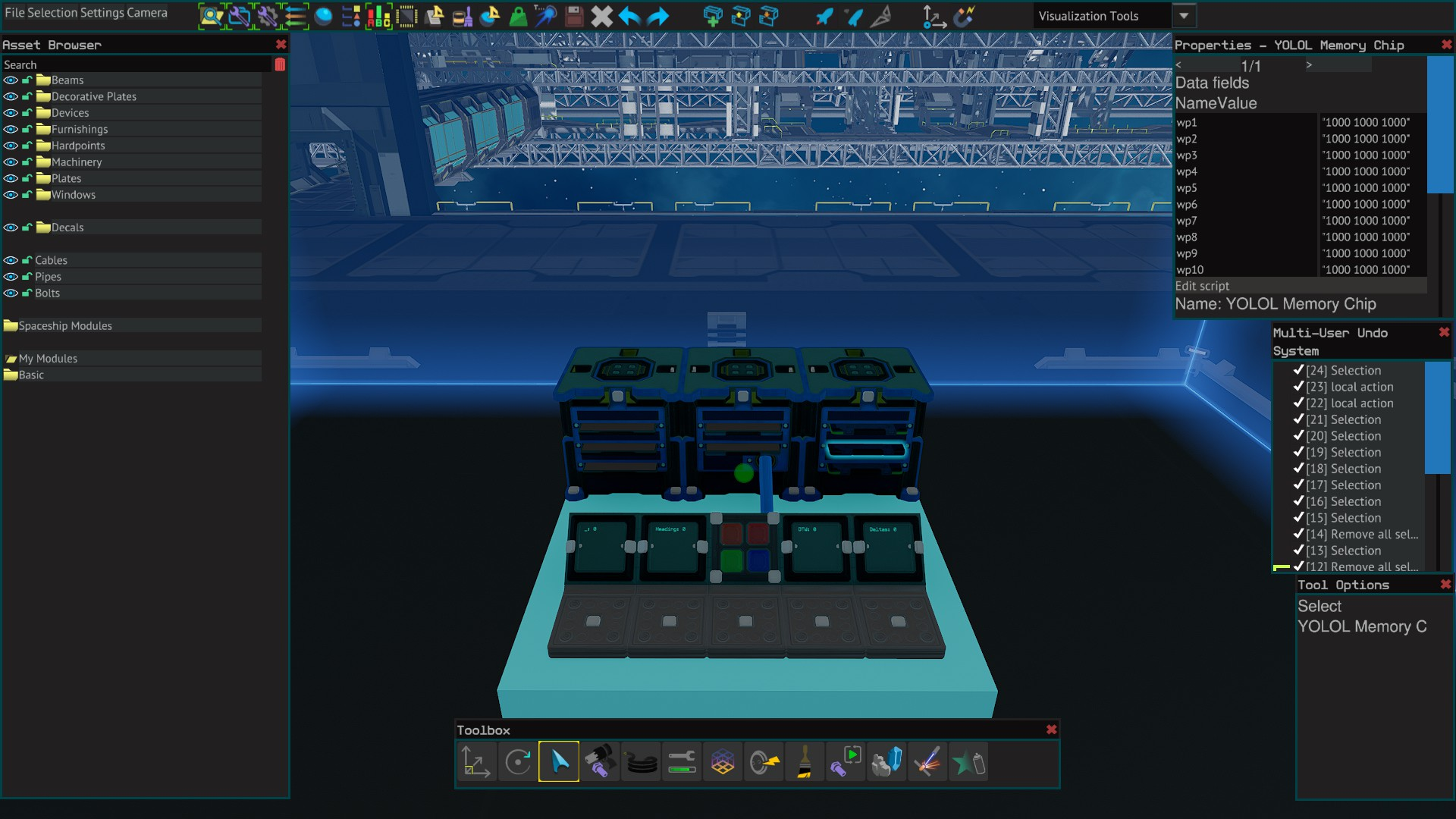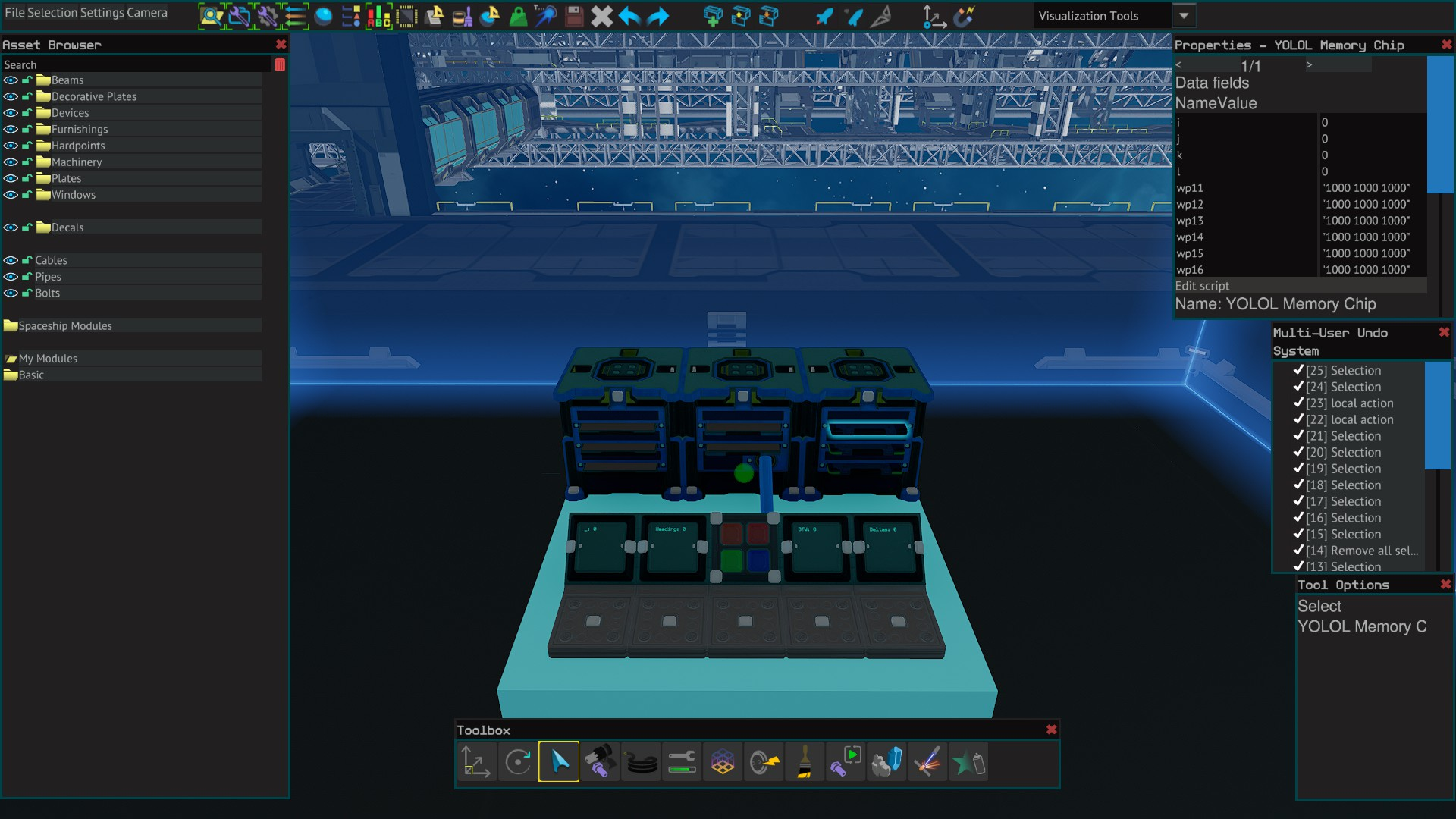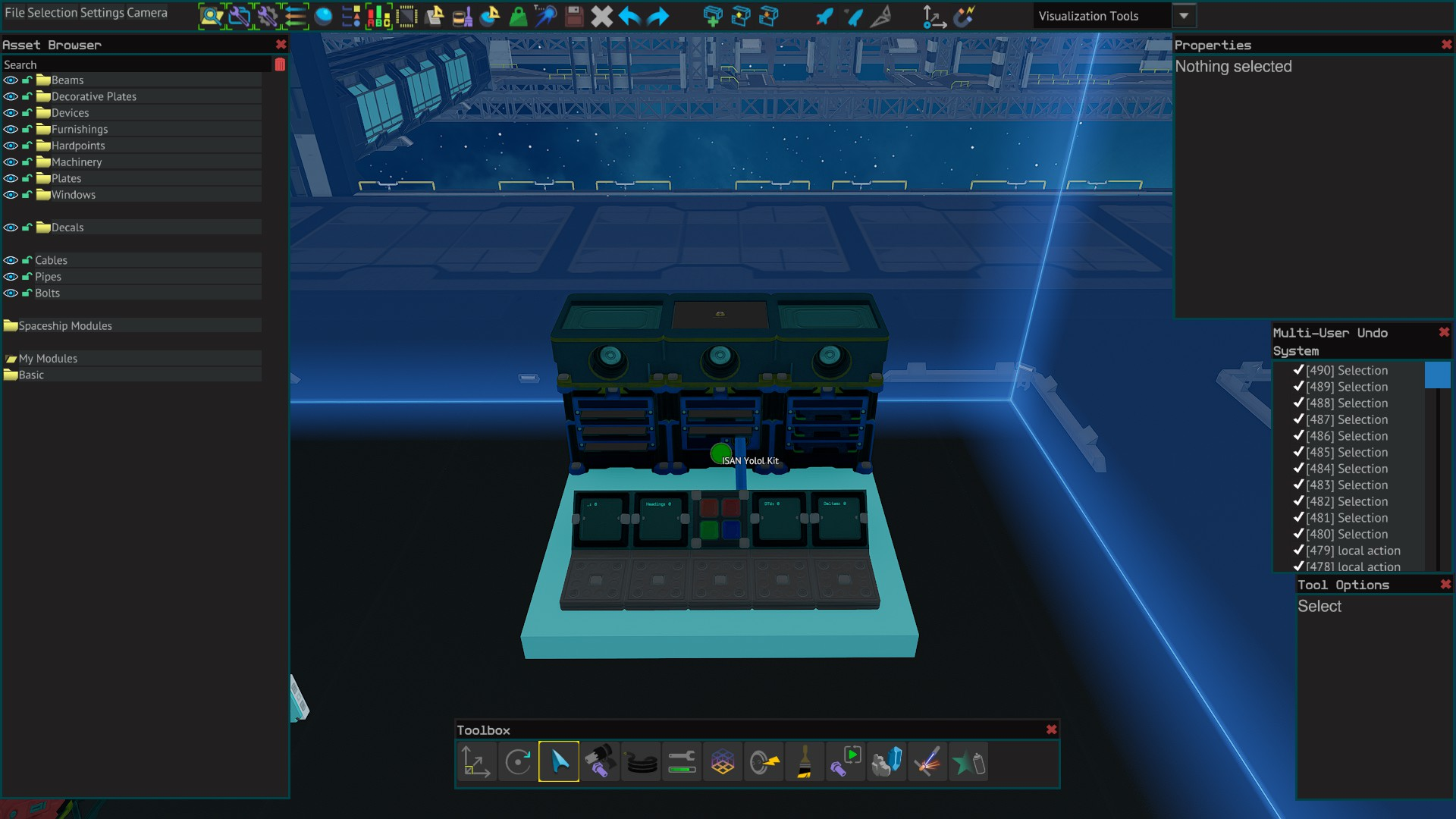An ISAN system with 16 programmable waypoints.

Includes; ship pitch and heading, waypoint pitch and heading, cycle through waypoints, save waypoints and a home waypoint feature.

Requires:

2x Basic YOLOL Chip

3x Memory Chip

3x YOLOL Rack 48x48x48cm

1x YOLOL Rack Chip Slot (2 slots)

2x YOLOL Rack Chip Slot (3 Slots)

4x Text Panel 24x24cm

4x Warning Light Button 12x12cm

YOLOL Code - copy & paste version:

A=1000 n=1000 pr=1 sp=1 div=10.4+2.4*pr so=1-sp o=160000 c=50 z="origin_" mr=z+"north" br=z+"south" cr=z+"east" z+="west" sm="\nS: " u=295384.615 k=-202105.263 l=-218947.368 mm=2*o e=8*o x/=so sm="" s="" rr="ISAN2 :_\n " xm="\nX: " ym="\nY: " zm="\nZ: " nn=1386666.667 ej=16*sp h="Q" pj=14-pr b=0.5 p=1000000 :at=mr :bt=br :ct=cr :dt=z x=0 t=p-:a t*=t i=p-:b i*=i g=p-:c g*=g f=p-:d f*=f x/=:a*:b*:c*:d goto14 h="M" t=p-:a t*=t er=(t-el)/4 el=t x/=:a :at=br x=8 gotopj i=p-:a i*=i fr=(i-fl)/4 fl=i x/=:a :at=cr x=9 gotopj g=p-:a g*=g gr=(g-gl)/4 gl=g x/=:a :at=z x=10 gotopj f=p-:a f*=f hr=(f-hl)/4 hl=f x/=:a :at=mr x=7 gotopj :_=rr+"\nSignal lost from:\n"+:at goto 11*(:a==0) st=:_!="" :_=rr+"\nReceiver(s) damaged." goto 12*st t+=er i+=fr g+=gr f+=hr x+=(6-x)*(x<8) :x=(t+i)/e+g/u+f/k :y=t/l+i/mm+(g+f)/nn :z=(g+f-t-i)/o st=:_=="" :_=rr+h+xm+:x/n*A+ym+:y/n*A+zm+:z/n*A+sm+s goto ej+x*so+p*st w+=:x uu+=:y vv+=:z r=w-tu j=uu-uv v=vv-vt ej+=(ii++%3)>1 gotox m=(r*r+j*j+v*v)^b s=m/div tu=w uv=uu vt=vv w=0 uu=0 vv=0 ej=16 :i=r :j=j :k=v :l=m :spd=s gotox :e="not in motion" :spd=0 goto x st=:_!="" :_=rr+"\n\n ISAN2\n OFFLINE" goto 20*st (ctrl+c=copy, ctrl+v=paste)

Waypoint Deltas - Advanced YOLOL Code:

YOLOL Code - copy & paste version:

a=:wpc k=100000 b="\n" :DTW=b+"Parsing Waypoint" wpn=:wpnum s="98743" i=0 o=0 j=-1 c=a c-=a-- d=5*(c>4)+2*(s>(s-c)) d+=c>d d+=c>d o+=d*10^j++ goto3+c<0 v=1 ifc=="-"thenv=-1 ifa!=""thena--endend i++ j=-1 :wZ=:wY :wY=:wX :wX=l l=o*v o=0 goto3+3*(i>3) :wpb=0 :wpi=0 :wpd=0 :wps=0 :wph=0 dx=:X-:wX dy=:Y-:wY dz=:Z-:wZ dx-=dx%1 dy-=dy%1 dz-=dz%1 x=dx^2 y=dy^2 z=dz^2 d=sqrt(x+y+z) ds=d ifds>k/10thends/=k ds*=100 ds+=" km"elseds-=ds%1 ds+=" m"end d1=180/3.142 s2=sqrt 0.5 //written by LizardFish xz=sqrt(dx^2+dz^2) q=abs(dx/xz) r=xz/d b1=-dy/d s1=-dz/xz p=(b1<s2)*(b1>-s2)*d1*(b1+b1^3/6) p+=(r<s2)*((dy<=0)*(90-d1*(r+r^3/6))+(dy>0)*(d1*(r+r^3/6)-90)) h=(q<=s2)*((dz<=0)*(d1*(q+q^3/6))+(dz>0)*(180-d1*(q+q^3/6))) h+=(s1<s2)*(s1>-s2)*(90-d1*(s1+s1^3/6)) ifdx>0thenh=360-h end h-=h%1 p-=p%1 goto17+(h!=360)*(p<91)*(p>-91) h="ERR" p="ERR" dtw="WP: "+wpn+b+"dX: "+dx+b+"dY: "+dy+b+"dZ: "+dz dtw+=b+"WH: "+h+" Deg"+b+"WP: "+p+" Deg"+b+ds :DTW=dtw if:wpb thengoto1end goto7 (ctrl+c=copy, ctrl+v=paste)

YOLOL Code - copy & paste version:

st="Live" b="\n" dx=:X-lx lx=:X ifdX==0thenst="Last Known" goto10end d1=180/3.142 s2=sqrt 0.5 //written by LizardFish xz=sqrt(:i^2+:k^2) q=abs(:i/xz) r=xz/:l c=1000 spd=:spd/c*c b1=:j/:l s1=:k/xz p=(b1<s2)*(b1>-s2)*d1*(b1+b1^3/6) p+=(r<s2)*((:j>=0)*(90-d1*(r+r^3/6))+(:j<0)*(d1*(r+r^3/6)-90)) h=(q<=s2)*((:k>=0)*(d1*(q+q^3/6))+(:k<0)*(180-d1*(q+q^3/6))) h+=(s1<s2)*(s1>-s2)*(90-d1*(s1+s1^3/6)) h-=h%1 p-=p%1 if:i<0thenh=360-h end h-=h%1 p-=p%1 goto9+(h!=360)*(p<91)*(p>-91) :Heading="Error\nRecalc" goto1 :Heading=b+h+" Deg"+b+"Pitch:"+b+p+" Deg"+b+"Spd:"+spd+b+st goto1 (ctrl+c=copy, ctrl+v=paste)

WayPoint Cycle - Basic YOLOL Code:

YOLOL Code - copy & paste version:

i=:wpi d=:wpd s=:wps h=:wph n=:wpnum+3 goto1+(i+d+s+h)>0 a=16 n+=(4-n)*h+i-d n+=a*(n<4)-a*(n>(a+3)) ifs==0thengoton end k=1000 wps=:x/k*k+" "+:y/k*k+" "+:z/k*k goton ifs then:wp1=wps end :wpc=:wp1 :wpb=1 :wpnum=n-3 goto20 ifs then:wp2=wps end :wpc=:wp2 :wpb=1 :wpnum=n-3 goto20 ifs then:wp3=wps end :wpc=:wp3 :wpb=1 :wpnum=n-3 goto20 ifs then:wp4=wps end :wpc=:wp4 :wpb=1 :wpnum=n-3 goto20 ifs then:wp5=wps end :wpc=:wp5 :wpb=1 :wpnum=n-3 goto20 ifs then:wp6=wps end :wpc=:wp6 :wpb=1 :wpnum=n-3 goto20 ifs then:wp7=wps end :wpc=:wp7 :wpb=1 :wpnum=n-3 goto20 ifs then:wp8=wps end :wpc=:wp8 :wpb=1 :wpnum=n-3 goto20 ifs then:wp9=wps end :wpc=:wp9 :wpb=1 :wpnum=n-3 goto20 ifs then:wp10=wps end :wpc=:wp10 :wpb=1 :wpnum=n-3 goto20 ifs then:wp11=wps end :wpc=:wp11 :wpb=1 :wpnum=n-3 goto20 ifs then:wp12=wps end :wpc=:wp12 :wpb=1 :wpnum=n-3 goto20 ifs then:wp13=wps end :wpc=:wp13 :wpb=1 :wpnum=n-3 goto20 ifs then:wp14=wps end :wpc=:wp14 :wpb=1 :wpnum=n-3 goto20 ifs then:wp15=wps end :wpc=:wp15 :wpb=1 :wpnum=n-3 goto20 ifs then:wp16=wps end :wpc=:wp16 :wpb=1 :wpnum=n-3 goto20 if:wpb thengoto20end goto1 (ctrl+c=copy, ctrl+v=paste)

Deltas - Basic YOLOL Code:

YOLOL Code - copy & paste version:

Memory Chip 1:

On Memory Chip 1, set the fields to:

X, Y, Z to 0, wx, wy, wz to 1000, wpc to "1000 1000 1000" (quotes included), wpnum to 1, spd to 0

Memory Chip 2:

On Memory Chip 2, set the fields to:

wp1 to wp10. Set the values to "1000 1000 1000" (quotes included)

Memory Chip 3:

On Memory Chip 3. set the fields to:

i, j, k, l to 0 and set fields wp11 to wp16 to "1000 1000 1000" (quotes included).

Installation

Place Small Navigation Receiver and change SignalStrenth to A, TargetMessage to AT and set the ListenAngle to 180. (page 2 of the fields)

Place 4x warning buttons, rename ButtonState field to wpi, wpd, wps, wph.

(Increment, Decrement, Save, Home), Rename all ButtonEnableBlink fields to wpb. ButtonStyle should be 1. ButtonColor is your choice. (I use wpi-orange, wpd-red, wps-green, wph-blue)

Place 4x text panels, rename PanelValue fields to _ (underscore), Deltas, DTW, Heading.

If the Heading screen says 'Live', its updating, if it says 'Last Known', you are not moving.

Wait for the blinking to stop after pressing a waypoint button.

Pressing the home waypoint button will take you straight to Waypoint 1.

ISAN Waypoint Yolol Kit

## Station - Map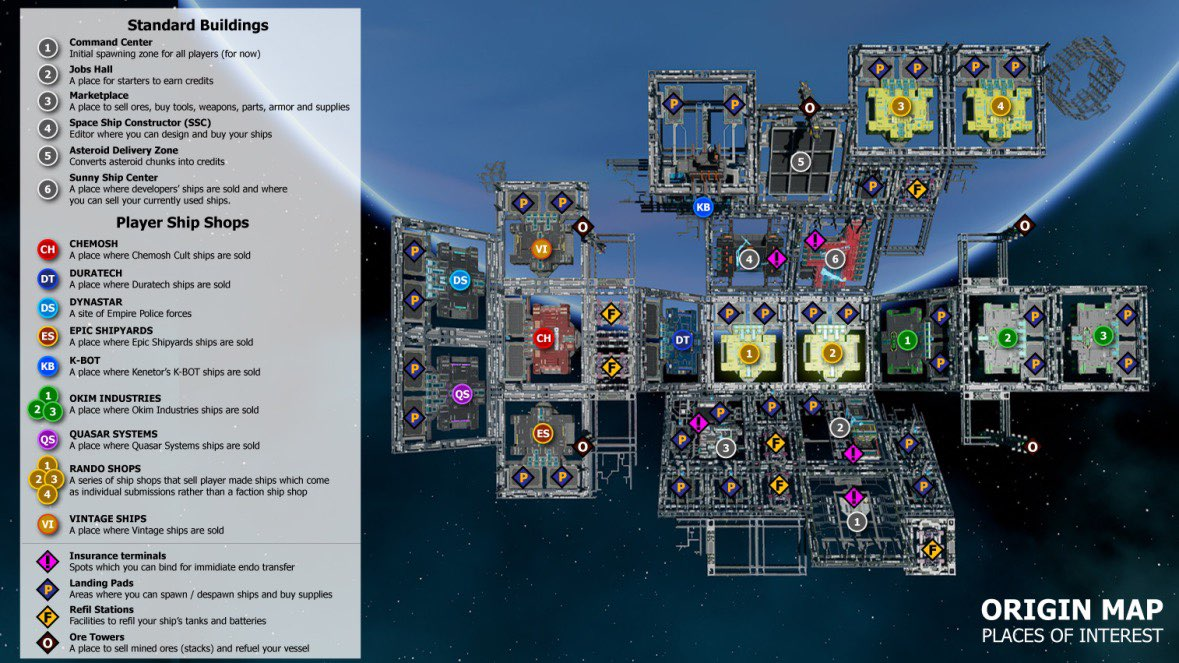Basic Station Map

No. 2 is now the Sunny Ship Shop.

You can sell unwanted ships at no. 6 Click spawn and wait a second or two and the spawn button will change to sell.

## EOS Belt - Map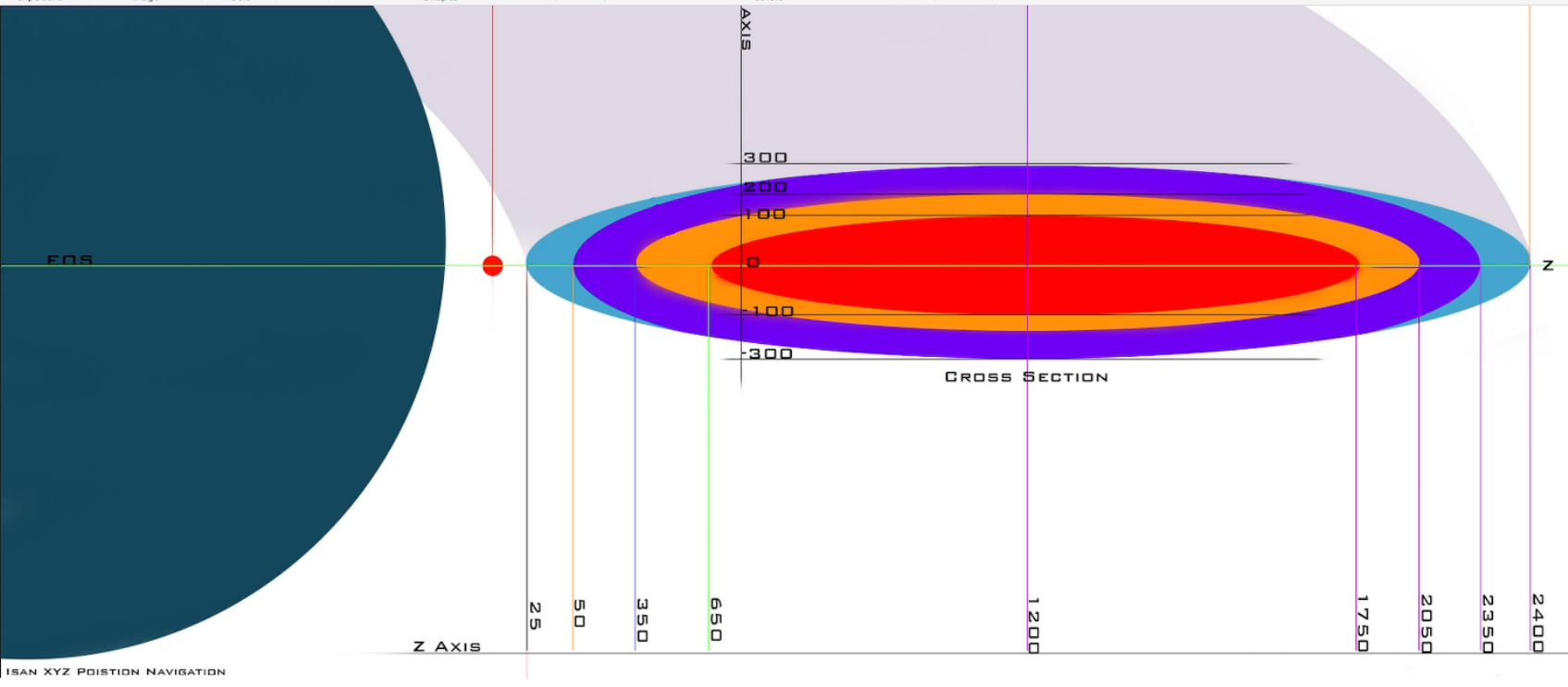Basic Belt Map

## Asteroid Location - Map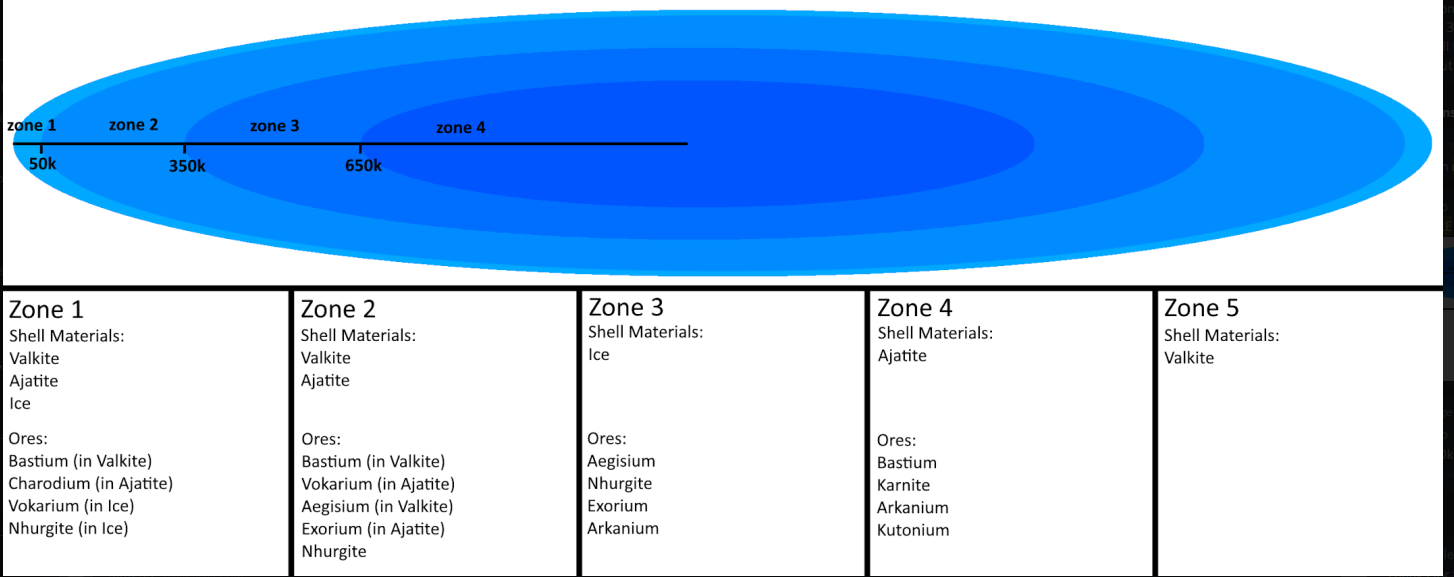Basic Asteroid Location Map

## (Template) - YOLOL Script

Script Title.

Requires:

YOLOL Code:

image

Advanced/Professional/Basic YOLOL Code - copy & paste version:

(ctrl+c=copy, ctrl+v=paste)

Installation

1

Yolol Kit

image

`Source: https://steamcommunity.com/sharedfiles/filedetails/?id=2580670649					`